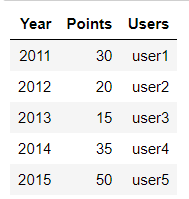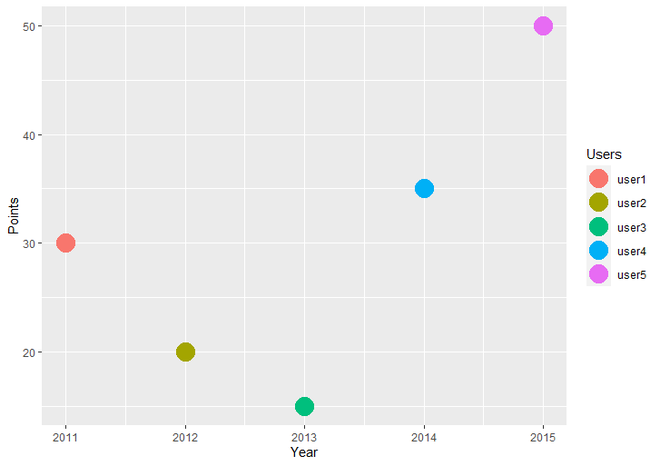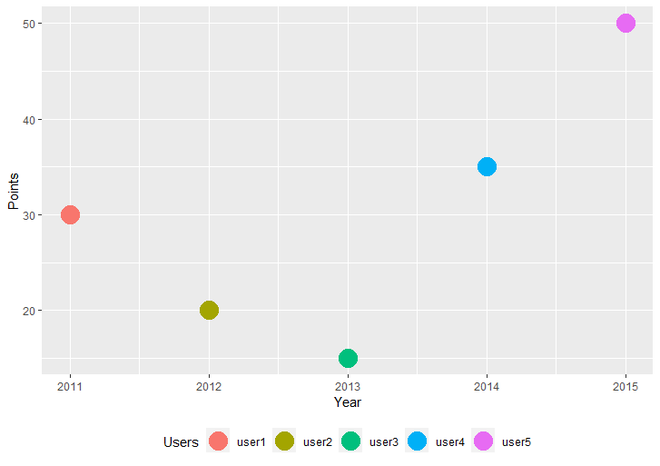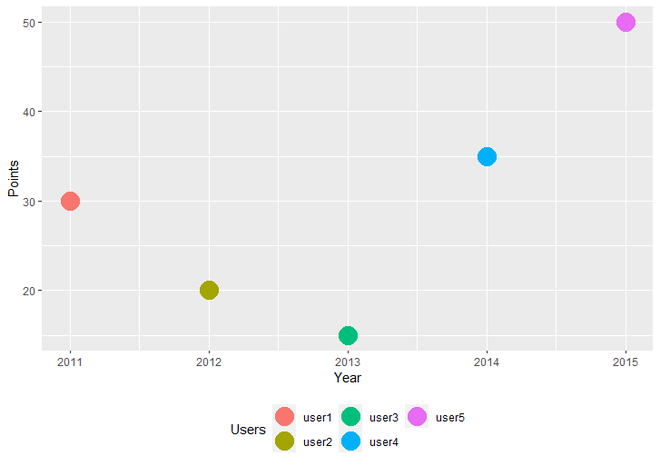# How to move a ggplot2 legend with multiple rows to the bottom of a plot in R

• Last Updated : 23 May, 2021

In this article, we are going to see how to draw ggplot2 legend at the bottom and with two Rows in R Programming Language. First, we have to create a simple data plot with legend. Here we will draw a Simple Scatter plot.

First, load the ggplot2 package by using library() function.

` library("ggplot2")`

Create a DataFrame for example. Here we create a simple DataFrame with three variables named Year, Points, and Users and then assign it to the data object.

## R

 `library``(``"ggplot2"``) ` ` `  `# Create a DataFrame ` `data <- ``data.frame``(Year = ``c``(2011, 2012, 2013, 2014, 2015), ` `                   ``Points = ``c``(30, 20, 15, 35, 50), ` `                   ``Users = ``c``(``"user1"``, ``"user2"``, ``"user3"``, ` `                             ``"user4"``, ``"user5"``)) ` `print``(data) `

Output:dataframe

For Create an R plot, we use ggplot() function and for make it scatter plot we add geom_point() function to ggplot() function. assign this whole plot to gplot data object.

Code:

## R

 `# Load Package ` `library``(``"ggplot2"``) ` ` `  `# Create a DataFrame  ` `data <- ``data.frame``(Year = ``c``(2011, 2012, 2013, 2014, 2015), ` `                   ``Points = ``c``(30, 20, 15, 35, 50), ` `                   ``Users = ``c``(``"user1"``, ``"user2"``, ``"user3"``,  ` `                             ``"user4"``, ``"user5"``)) ` ` `  `# Create a Scatter Plot and assign it  ` `# to gplot data object ` `gplot <- ``ggplot``(data, ``aes``(Year, Points, color = Users)) +    ` `  ``geom_point``(size = 7) ` `gplot `

Output:Simple Scatter Plot with legend

## Draw ggplot2 Legend at the Bottom of plot

To draw ggplot2 legend at the bottom of the plot, we simply add the theme() function to geom_point() function.

Syntax : theme(legend.position)

Parameter : In General, theme() function has many parameters to specify the theme of the plot but here we use only legend.position parameter which specify the position of Legend.

Return : Theme of the plot.

We can specify the value of legend.position parameter from left, right, top and bottom. To draw legend at bottom of graph, we use ‘bottom’ as a value of legend.position parameter.

## R

 `# Load Package ` `library``(ggplot2) ` ` `  `# Create a DataFrame For plot ` `data <- ``data.frame``(Year = ``c``(2011, 2012, 2013, 2014, 2015), ` `                   ``Points = ``c``(30, 20, 15, 35, 50), ` `                   ``Users = ``c``(``"user1"``, ``"user2"``, ``"user3"``,  ` `                             ``"user4"``, ``"user5"``)) ` ` `  `# Create a simple scatter plot  ` `# with legend at bottom. ` `ggplot``(data, ``aes``(Year, Points, color = Users)) +    ` `  ``geom_point``(size = 7)+ ` `  ``theme``(legend.position = ``"bottom"``) `

Output:Scatter Plot with Legend at bottom

## Draw ggplot2 Legend at the bottom with Two Rows

If we want to draw ggplot2 Legend at the bottom of the plot with two rows, we have to add guides and guide_legend functions to the theme() function. Inside guides() function, we take parameter named color, which has call to guide_legend() guide function as value. Inside guide_legend() function, we take an argument called nrow, which has the desired number of rows of legend as value.

Syntax : guide_legend(nrow)

Parameter :

• nrow : The Desired Number of rows of legend.

Return : Legend Guides for various scales

Code:

## R

 `# Load Package ` `library``(ggplot2) ` ` `  `# Create a DataFrame For plot ` `data <- ``data.frame``(Year = ``c``(2011, 2012, 2013, 2014, 2015), ` `                   ``Points = ``c``(30, 20, 15, 35, 50), ` `                   ``Users = ``c``(``"user1"``, ``"user2"``, ``"user3"``, ` `                             ``"user4"``, ``"user5"``)) ` ` `  `# Create a simple scatter plot with ` `# legend at bottom and with Two Rows. ` `# Specifies the Number of legend Rows ` `ggplot``(data, ``aes``(Year, Points, color = Users)) +    ` `  ``geom_point``(size = 7)+ ` `  ``theme``(legend.position = ``"bottom"``)+ ` `  ``guides``(color = ``guide_legend``(nrow = 2))       `

Output:Scatter plot with Legend at bottom with Two Rows

My Personal Notes arrow_drop_up
Recommended Articles
Page :